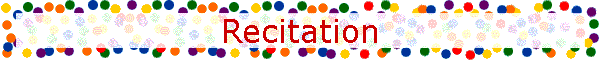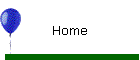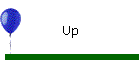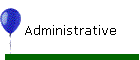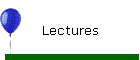Introduction to Engineering and Technology1st week Topics: A proposed resolution process for applied problems in Physics and Mathematics   Basic Points: STEP 1: Identify the quantity or quantities the problem asks for. STEP 2: Identify an expression that provides the unknown quantity. STEP 3: If the expression has to the left the unknown quantity and to the right all known quantities you have the general expression that gives you the quantity asked for. If the expression has to the right an unknown quantity, write another formula that provides the unknown quantity in terms of other quantities. Continue the process until all quantities to the right are known. Step 4: Now you have the general expression that gives you the quantity asked for in symbolic form. Step 5: Graph of the general solution Step 6: Now substitute the numerical values along with the unit of measurement. (If a numerical answer is required.) Step 7: Perform the operation with the numerical values and units. Report the result in the form numerical value with unit. Note the three step (three lines resolution process from the general expression to the numerical result.) Step 8: Investigation of the final solution (Step 4 and 7)    Examples:   Applications:   Homework:   References:Basic Points: Using computer algebra packages (Mathematica, Matlab, MathCad)   Examples:   Applications:   Homework:   References:Basic Points: Homework Format Guidelines   1. Homework is to be turned in on Letter size (8½*11) plain paper. Please do not use any other kind of paper.   2. Clearly print student’s [Student number], [First Name], [Last Name] on top of the first page. Please use the name exactly as shown on the class roll.   3. Homework should be stapled. Please do not glue, clip, or fold your homework. Loose pages will not be accepted.   4. Homework should be done on one side of paper only. Writing should be legible.  The steps should be clear if would like the grader to understand the process of your thoughts.   5. If there are sub-problems in one homework problem, answers to each sub-problem should be worked on in order, separately. Physical quantities have units of measurements, include them.   6. Homework should be done using current technology tools.   All guidelines should be followed exactly for grading accuracy and efficiency. Your cooperation will certainly be appreciated!   Examples:   Applications:   Homework:   References: 2nd week Topics: Vector Algebra Addition - Subtraction Inner multiplication - Outer multiplication   Coordinate Systems Cartesian Circular Cylindrical Spherical Element of length Element of surface Element of volume   Vector calculus Plot of: Scalar functions Vector functions iso- lines Gradient of a function Divergence of a function Rotation of a function   Methodology:   Basic Points:   Examples:   Applications:   Review Questions:   Homework:   References: 3th week Solution of Electromagnetic theory problems Methodology: Techniques for the solution of problems    Topics:   Basic Points:   Examples:   Applications:   Homework:   References: 4th week Topics:   Methodology:   Basic Points:   Examples:   Applications:   Homework:   References: 5th week Solution of Electrostatics and Quazi - electrostatics problems Topics:   Methodology:   Basic Points:   Examples:   Applications:   Homework:   References: 6th week Topics:   Methodology:   Basic Points:   Examples:   Applications:   Homework:   References: 7th week Topics:   Methodology:   Basic Points:   Examples:   Applications:   Homework:   References: 8th week Topics:   Methodology:   Basic Points:   Examples:   Applications:   Homework:   References: 9th week Solution of Magnetostatics and Quazi - magnetostatics problems Topics:   Methodology:   Basic Points:   Examples:   Applications:   Homework:   References: 10th week Topics:   Methodology:   Basic Points:   Examples:   Applications:   Homework:   References: 11th week Topics:   Methodology:   Basic Points:   Examples:   Applications:   Homework:   References: 12th week Topics:   Methodology:   Basic Points:   Examples:   Applications:   Homework:   References: 13th week Topics:   Methodology:   Basic Points:   Examples:   Applications:   Homework:   References: 14th week Topics:   Methodology:   Basic Points:   Examples:   Applications:   Homework:   References: 15th week Review:   Topics:   Methodology:   Basic Points:   Examples:  Applications:   Homework:   References: 16th week Final examination